Wir verwenden Google für unsere Suche. Mit Klick auf „Suche aktivieren“ aktivieren Sie das Suchfeld und akzeptieren die Nutzungsbedingungen.

# New in SG-Lib 2.7

###### ipcam_2014b(n,url)- Returns images of an IP camera such as an iPhone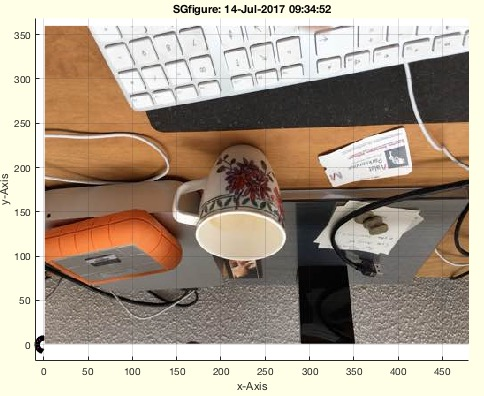% ipcam_2014b(n,url) - Returns images of an IP camera such as an iPhone% (by Tim Lueth, VLFL-Lib, 2015-OKT-10 as class: EXPERIMENTS)% % =======================================================================% OBSOLETE (2017-07-11) - USE FAMILY 'ipcam' INSTEAD% =======================================================================% % ipcam works inside of the EUDOROAM Network using the accounts of type: % gu28kix@eduroam.mwn.de. % >>> It is possible to activate the WLAN connection of the MAC computer % in addition to the Ethernet cable connection and have additional IP % address areas. % >>> The ipcam Application can be downloaded for iPhone in the App-Store % (Status of: 2017-03-23)% % Introduced first in SolidGeometry 2.7% % ss=ipcam_2014b([n,url])% === INPUT PARAMETERS ===% n: number of desired mouse clicks to stop% url: url of ip cam server (iphone etc.)% === OUTPUT RESULTS ======% ss: image% % EXAMPLE: Create a stereo image% fh=ipcam; X1=fh.CData;% fh=ipcam; X2=fh.CData;% I1=rgb2gray(X1); I2=rgb2gray(X2); % points1 = detectHarrisFeatures(I1); points2 = detectHarrisFeatures(I2);% %
###### FLofCVL(VL,ni,at,tt)- returns the faces for a tube-style vertex list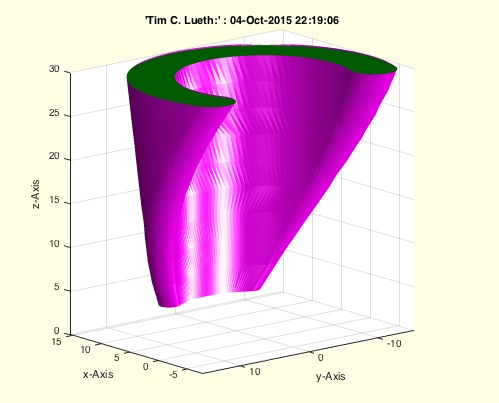% FLofCVL(VL,ni,at,tt) - returns the faces for a tube-style vertex list% (by Tim Lueth, VLFL-Lib, 2015-OKT-04 as class: SURFACES)% % This procedure is used in SGsphere. Does work with one PL. Analyzes % itself the z-values.% The procedure expects a sorted vertex list consisting of several % succeeding polygon point list with different z-values. If just the VL % is input argument, the procedure analyzes the z-values and reconstructs % based on same z-values different levels and creates the outer walls % between those polygons. (Status of: 2015-10-04)% % [FLB,FLW,FLT,CiL,ni]=FLofCVL(VL,[ni,at,tt])% === INPUT PARAMETERS ===% VL: Vertex list% ni: Optional size list% at: optional assignment type; default is 'number'% tt: optional turning type; default is 'none'% === OUTPUT RESULTS ======% FLB: Facet list of the walls% FLW: Facet list of the bottom% FLT: Facet list of the cover% CiL: CiL% ni: size list% % EXAMPLE: % VL=[]; for i=1:10; VL=[VL;VLaddz(PLstar(10,10),3*i)]; end; FLofCVL(VL);%
###### SGsphere(R,nr,wt,wb,Ry,Rz)- returns a spherical SG with minimal nr of points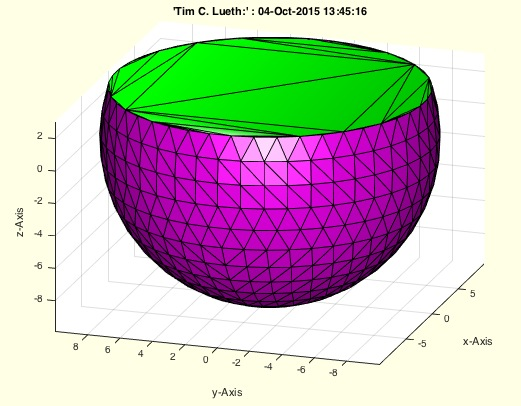% SGsphere(R,nr,wt,wb,Ry,Rz) - returns a spherical SG with minimal nr of points% (by Tim Lueth, VLFL-Lib, 2015-OKT-04 as class: SURFACES)% % returns a sphere or an ellipsoid, depending on the given parameters % (Status of: 2017-04-17)% % See also: SGcone, SGbox, SGlinkage, SGtext, SGspherelink% % [SG,FLW,FLB,FLT]=SGsphere(R,[nr,wt,wb,Ry,Rz])% === INPUT PARAMETERS ===% R: Radius% nr: number of points; default is [];% wt: top angle; default is +pi/2% wb: bottom angle; default is -pi/2% Ry: Radius in Y; default is R% Rz: Radius in Z; default is R% === OUTPUT RESULTS ======% SG: Solid Geometry% FLW: Faces of the wall, related to SG.VL% FLB: Faces of the bottom, related to SG.VL% FLT: Faces of the cover, related to SG.VL% % EXAMPLE: % SGsphere(10,[],pi/10)%
###### isint32(a)- returns true or false if a number has no fraction% isint32(a) - returns true or false if a number has no fraction% (by Tim Lueth, VLFL-Lib, 2015-OKT-04 as class: AUXILIARY PROCEDURES)% % In contrast to: isequal((rem(a,1),0)% this procedure is faster and automatically detects the limit of int32.% % Can be used for calculation of number of egdes: if ~isint32(nf); % nf=nofrd(R,nf/2); end;% % (Status of: 2015-10-04)% % b=isint32(a)% === INPUT PARAMETERS ===% a: number% === OUTPUT RESULTS ======% b: true if isequal((rem(a,1),0) %
###### int32orfloat(a)- changes type to integer float is an integer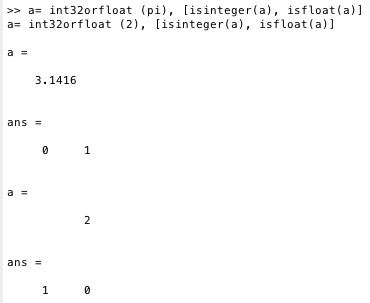% int32orfloat(a) - changes type to integer float is an integer% (by Tim Lueth, VLFL-Lib, 2015-OKT-04 as class: AUXILIARY PROCEDURES)% % this procedure can be used to distinguish for nofrd wether the argument % is a desired point number or a desired resolution of an object.% if (mod(a,(1-1e-18))<1e-9); a=int32(a); end; [isinteger(a), isfloat(a)]% (Status of: 2015-10-04)% % a=int32orfloat(a)% === INPUT PARAMETERS ===% a: float % === OUTPUT RESULTS ======% a: same number as float or as integer%
###### size2index(ni)- converts a size list into an index list [start end]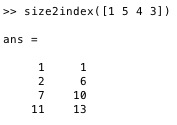% size2index(ni) - converts a size list into an index list [start end]% (by Tim Lueth, VLFL-Lib, 2015-OKT-04 as class: AUXILIARY PROCEDURES)% % The size list contains element sizes [n1 n2 n3 n4 n5..]% The index list contains elements of type [startindex endindex]% Backwards it is: index2size = il(:,2)-il(:,1)+1 (Status of: 2015-10-04)% % il=size2index(ni)% === INPUT PARAMETERS ===% ni: list of [size of element]% === OUTPUT RESULTS ======% il: list of [startindex endindex]% % EXAMPLE: % size2index([1 3 4 3])%
###### PLminyx(PL,maxY)- returns the point with minimal y and x value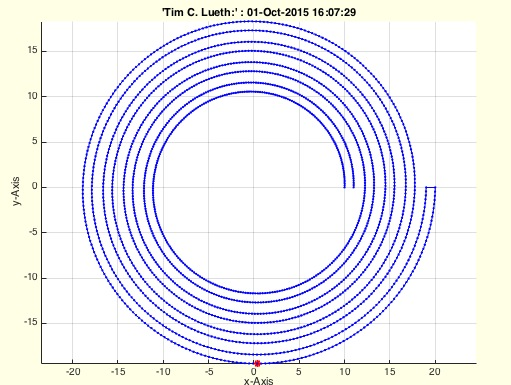% PLminyx(PL,maxY) - returns the point with minimal y and x value% (by Tim Lueth, VLFL-Lib, 2015-OKT-01 as class: AUXILIARY PROCEDURES)% % See also: PLminangle% % [P,pi]=PLminyx(PL,[maxY])% === INPUT PARAMETERS ===% PL: Point Liste% maxY: if true; we search for maximum y and maximum x% === OUTPUT RESULTS ======% P: Point with minimal y and minimal x% pi: find index in PL% % EXAMPLE: % PLminyx(CPLspiral(10,20,8*pi))% PLminyx(CPLspiral(10,20,8*pi),true)% [~,ci]=PLminyx(CPLB); CPLB=circshift(CPLB,-(ci-1));%
###### exp_2015_09_30- EXPERIMENT for a sphere with minimal number of points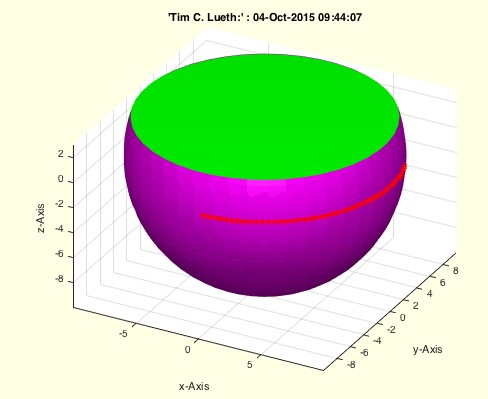% exp_2015_09_30 - EXPERIMENT for a sphere with minimal number of points% (by Tim Lueth, VLFL-Lib, 2015-SEP-30 as class: SURFACES)% % In this experiment, % 1st a shape curve is calculated for the sphere% 2nd for each z-value a circle with minimal number of points is created % to create a vertex list% 3rd between two circles the walls are calculated using SGof2CPL% 4th floor and Top faces are added (Status of: 2015-10-04)% % exp_2015_09_30%
###### exp_2015_09_29- EXPERIMENT to show the generation of Laser-Cutter SVGs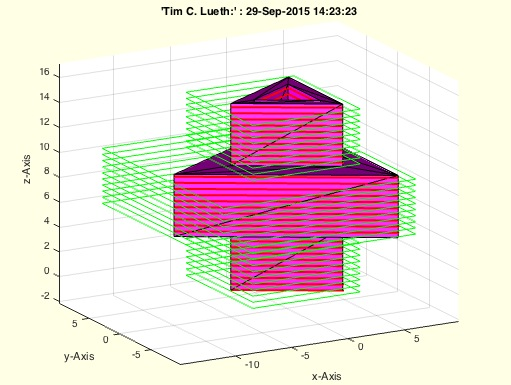% exp_2015_09_29 - EXPERIMENT to show the generation of Laser-Cutter SVGs% (by Tim Lueth, VLFL-Lib, 2015-SEP-29 as class: EXPERIMENTS)% % exp_2015_09_29%
###### CPLofBB(BB,d)- returns a CPL around a 2D Bounding Contour BB% CPLofBB(BB,d) - returns a CPL around a 2D Bounding Contour BB% (by Tim Lueth, VLFL-Lib, 2015-SEP-29 as class: CLOSED POLYGON LISTS)% % Used for creating a surrounding BB for a CPL. (Status of: 2017-01-05)% % See also: BBofCPL, BBiscollofVL, BBofVL, SGofBB, VLFLofBB, % outboundingbox, BBofSG% % CPL=CPLofBB(BB,[d])% === INPUT PARAMETERS ===% BB: Bounding Box or CPL% d: distance around the bounding box; default is 1% === OUTPUT RESULTS ======% CPL: Closed Polygon Liste% % EXAMPLE: % CPLofBB(CPLslicer(SGsample(7),9))%
###### CPLofSGslice(SG,z)- returns the CPL of a sliced SG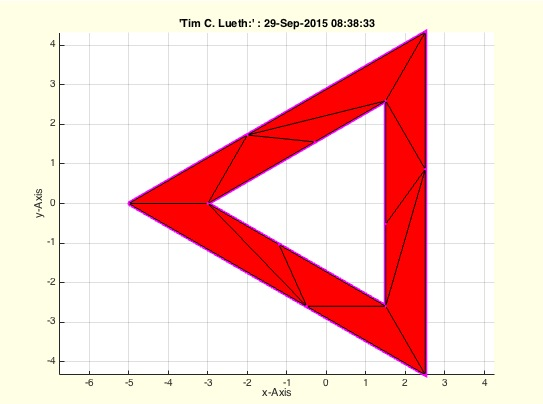% CPLofSGslice(SG,z) - returns the CPL of a sliced SG% (by Tim Lueth, VLFL-Lib, 2015-SEP-29 as class: SLICES)% % [CPL,warn]=CPLofSGslice(SG,z)% === INPUT PARAMETERS ===% SG: Solid Geoemtry% z: z value for slicing% === OUTPUT RESULTS ======% CPL: Closed Polygon List% warn: % % EXAMPLE: % CPLofSGslice(SGsample(7),3)%
###### iscrossingCPL(CPLA,CPLB,touch)- checks crossing/touching of two CPLs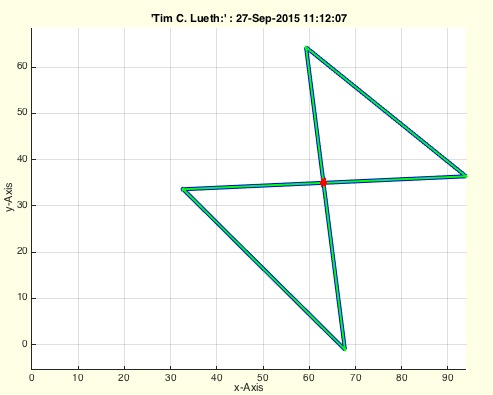% iscrossingCPL(CPLA,CPLB,touch) - checks crossing/touching of two CPLs% (by Tim Lueth, VLFL-Lib, 2015-SEP-27 as class: CLOSED POLYGON LISTS)% % Not optimized procedure for use/help during development.% (Status of: 2015-09-27)% % [c,pc]=iscrossingCPL(CPLA,[CPLB,touch])% === INPUT PARAMETERS ===% CPLA: Closed polygon A% CPLB: Closed polygon B% touch: check touch instead of cross; default is true% === OUTPUT RESULTS ======% c: true/false% pc: first detected crossing point%
###### exp_2015_09_27- EXPERIMENT for systematically testing SGofCPL2z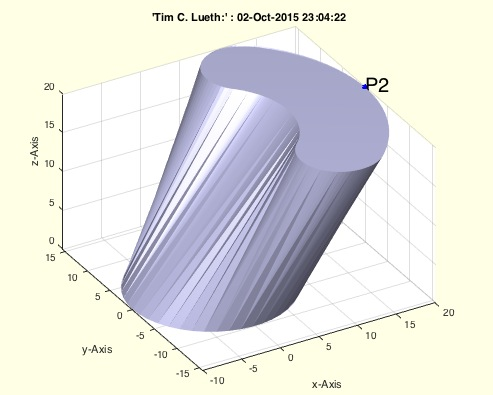%% PUBLISHABLE EXP_2015_09_27 EXPERIMENT FOR SYSTEMATICALLY TESTING SGOFCPL2Z% (by Tim Lueth, VLFL-Lib, 2015-SEP-27 as class: EXPERIMENTS)%%% exp_2015_09_27 - EXPERIMENT for systematically testing SGofCPL2z% (by Tim Lueth, VLFL-Lib, 2015-SEP-27 as class: EXPERIMENTS)% % exp_2015_09_27%
###### CPLremnoise(CPL,r,maxw)- returns a smoother CPL% CPLremnoise(CPL,r,maxw) - returns a smoother CPL% (by Tim Lueth, VLFL-Lib, 2015-SEP-25 as class: ANALYTICAL GEOMETRY)% % Better name would be CPLremDetails% This procedure removes 'details' between two points on a closed contour % point list (CPL). Such 'details' or 'noise' can appear after merging a % half circle with a square. Suddenly a zig-zag step is part of a contour % that should have no sharp edges.% r spezifies the maximum length between 2 points% maxw spezifies the minimum angle to remove points% if maxw==0; % (Status of: 2016-01-02)% % CPLN=CPLremnoise(CPL,[r,maxw])% === INPUT PARAMETERS ===% CPL: CPL% r: minimal allowed distance between 2 points; 0.05;% maxw: noise angle; default is pi/2 -1e-5;% === OUTPUT RESULTS ======% CPLN: CPLwithout jitter% % EXAMPLE: try:% PL=PLradialEdges(CPLspiral(10,20,2*pi,1)); CPLremnoise(PL,1)% C=CPLremnoise(C,sofrd(r)+1e-5,(2*pi+1e-5)/nofrd(r));% % %
###### CPLcopypatternPL(CPL,PL,dw)- copies a CPL at positions of a PL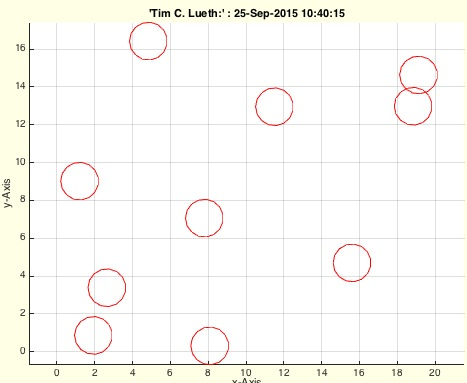% CPLcopypatternPL(CPL,PL,dw) - copies a CPL at positions of a PL% (by Tim Lueth, VLFL-Lib, 2015-SEP-25 as class: CLOSED POLYGON LISTS)% % CPLN=CPLcopypatternPL(CPL,PL,[dw])% === INPUT PARAMETERS ===% CPL: CPL to copy (nx2)% PL: PL of relative Positions (nx2)% dw: optional turing angle or turning array [nx1]; default is 0% === OUTPUT RESULTS ======% CPLN: Final CPL [nx2]% % EXAMPLE: % CPLcopypatternPL(PLcircle(1),20*rand(10,2))% CPLcopypatternPL(PLcircle(1,4),20*rand(10,2),pi/10)%
###### CPLspiral(RI,RO,wm,d,n)- returns a closed polygon in spiral shape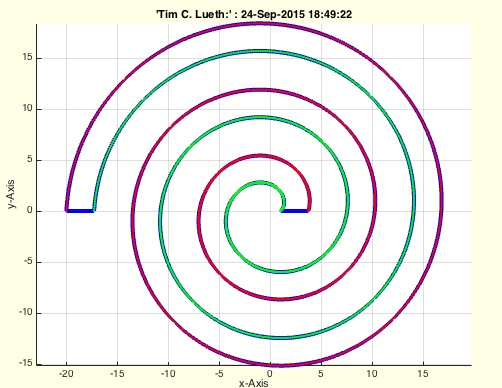% CPLspiral(RI,RO,wm,d,n) - returns a closed polygon in spiral shape% (by Tim Lueth, VLFL-Lib, 2015-SEP-24 as class: CLOSED POLYGON LISTS)% % PL=CPLspiral(RI,RO,[wm,d,n])% === INPUT PARAMETERS ===% RI: Inner radius% RO: Outer radius% wm: turning angle; default is 2pi% d: wall thickness; default is (RO-RI)/2/(wm/(2*pi)+1% n: number of points; default is nofrd% === OUTPUT RESULTS ======% PL: Point list [nx2]%
###### PLspiral(RI,RO,wm,n)- return the PL of a spiral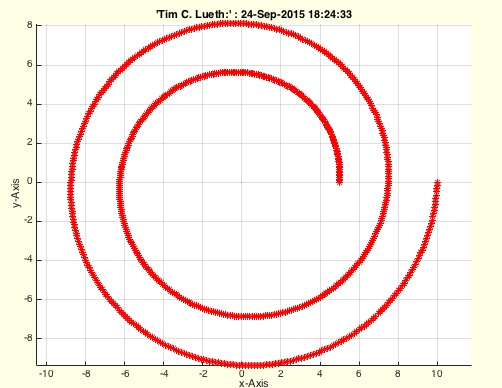% PLspiral(RI,RO,wm,n) - return the PL of a spiral% (by Tim Lueth, VLFL-Lib, 2015-SEP-24 as class: ANALYTICAL GEOMETRY)% % See also: PLcircle, PLcircseg, PLevolvente, PLgear, PLhelix, PLkidney, % PLrand, PLsquare, PLstar% % PL=PLspiral(RI,RO,[wm,n])% === INPUT PARAMETERS ===% RI: Inner Diameter% RO: Outer Diameter% wm: Desired angle; default is 2pi% n: number of points; default is nofrd((RO+RI)/2)/2pi*wm% === OUTPUT RESULTS ======% PL: Point list [nx2]% % EXAMPLE: Two turn spiral% PLspiral(5,10,4*pi)%
###### PLangle2(PL)- returns the angles of a points list% PLangle2(PL) - returns the angles of a points list% (by Tim Lueth, VLFL-Lib, 2015-SEP-24 as class: ANALYZING PROCEDURES)% % In contrast to VLangle, PLangle uses crossz to calculate an % orientation: Positive values are mathematical positive (right hand). % (Status of: 2015-09-22)% % [w,d,nw,wi]=PLangle2(PL)% === INPUT PARAMETERS ===% PL: vector list% === OUTPUT RESULTS ======% w: angle list% d: distance list% nw: % wi: % % EXAMPLE: % [PLstar(10,10) PLangle(PLstar(10,10))/pi*180]% %
###### PLcrossCPLline(CPL,pa,pb,touch,full)- return the crossing point of a CPL and a line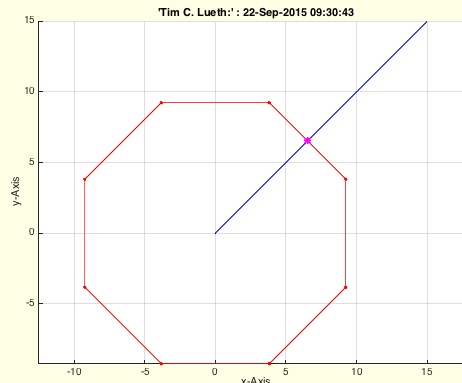% PLcrossCPLline(CPL,pa,pb,touch,full) - return the crossing point of a % CPL and a line% (by Tim Lueth, VLFL-Lib, 2015-SEP-22 as class: ANALYTICAL GEOMETRY)% % Checks on crossings or touch (selected by parameter).% In future can deliver the full crossing list on demand (Status of: % 2015-09-22)% % [CP,k,k1]=PLcrossCPLline(CPL,pa,pb,[touch,full])% === INPUT PARAMETERS ===% CPL: Closed Polygon List% pa: line start point% pb: line end point% touch: check on touch instead of cross; default is false% full: return a full crossing point list; default is false% === OUTPUT RESULTS ======% CP: Crossing Point% k: index in PL% k1: subsequent index in PL% % EXAMPLE: % PLcrossCPLline(PLcircle(10,8),[0 0 ],[15 15])%